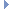OpenMM
DrudeLangevinIntegrator Class Reference

This Integrator simulates systems that include Drude particles. More...Inheritance diagram for DrudeLangevinIntegrator:

List of all members.

## Public Member Functions

def getTemperature
getTemperature(self) -> double
def setTemperature
Set the temperature of the main heat bath (in Kelvin).
def getFriction
getFriction(self) -> double
def setFriction
Set the friction coefficient which determines how strongly the system is coupled to the main heat bath (in inverse ps).
def getDrudeTemperature
getDrudeTemperature(self) -> double
def setDrudeTemperature
Set the temperature of the heat bath applied to internal coordinates of Drude particles (in Kelvin).
def getDrudeFriction
getDrudeFriction(self) -> double
def setDrudeFriction
Set the friction coefficient which determines how strongly the internal coordinates of Drude particles are coupled to the heat bath (in inverse ps).
def getMaxDrudeDistance
getMaxDrudeDistance(self) -> double
def setMaxDrudeDistance
Set the maximum distance a Drude particle can ever move from its parent particle, measured in nm.
def getRandomNumberSeed
getRandomNumberSeed(self) -> int
def setRandomNumberSeed
Set the random number seed.
def step
Advance a simulation through time by taking a series of time steps.
def __init__
__init__(self, temperature, frictionCoeff, drudeTemperature, drudeFrictionCoeff, stepSize) -> DrudeLangevinIntegrator __init__(self, other) -> DrudeLangevinIntegrator

this

## Detailed Description

This Integrator simulates systems that include Drude particles.

It applies two different Langevin thermostats to different parts of the system. The first is applied to ordinary particles (ones that are not part of a Drude particle pair), as well as to the center of mass of each Drude particle pair. A second thermostat, typically with a much lower temperature, is applied to the relative internal displacement of each pair.

This integrator can optionally set an upper limit on how far any Drude particle is ever allowed to get from its parent particle. This can sometimes help to improve stability. The limit is enforced with a hard wall constraint.

This Integrator requires the System to include a DrudeForce, which it uses to identify the Drude particles.

## Constructor & Destructor Documentation

 def __init__ ( self, args )

__init__(self, temperature, frictionCoeff, drudeTemperature, drudeFrictionCoeff, stepSize) -> DrudeLangevinIntegrator __init__(self, other) -> DrudeLangevinIntegrator

Create a DrudeLangevinIntegrator.

Parameters:
 temperature (double) the temperature of the main heat bath (in Kelvin) frictionCoeff (double) the friction coefficient which couples the system to the main heat bath (in inverse picoseconds) drudeTemperature (double) the temperature of the heat bath applied to internal coordinates of Drude particles (in Kelvin) drudeFrictionCoeff (double) the friction coefficient which couples the system to the heat bath applied to internal coordinates of Drude particles (in inverse picoseconds) stepSize (double) the step size with which to integrator the system (in picoseconds)

## Member Function Documentation

 def getDrudeFriction ( self )

getDrudeFriction(self) -> double

Get the friction coefficient which determines how strongly the internal coordinates of Drude particles are coupled to the heat bath (in inverse ps).

Returns:
(double) the friction coefficient, measured in 1/ps
 def getDrudeTemperature ( self )

getDrudeTemperature(self) -> double

Get the temperature of the heat bath applied to internal coordinates of Drude particles (in Kelvin).

Returns:
(double) the temperature of the heat bath, measured in Kelvin
 def getFriction ( self )

getFriction(self) -> double

Get the friction coefficient which determines how strongly the system is coupled to the main heat bath (in inverse ps).

Returns:
(double) the friction coefficient, measured in 1/ps
 def getMaxDrudeDistance ( self )

getMaxDrudeDistance(self) -> double

Get the maximum distance a Drude particle can ever move from its parent particle, measured in nm. This is implemented with a hard wall constraint. If this distance is set to 0 (the default), the hard wall constraint is omitted.

 def getRandomNumberSeed ( self )

getRandomNumberSeed(self) -> int

Get the random number seed. See setRandomNumberSeed() for details.

 def getTemperature ( self )

getTemperature(self) -> double

Get the temperature of the main heat bath (in Kelvin).

Returns:
(double) the temperature of the heat bath, measured in Kelvin
 def setDrudeFriction ( self, coeff )

Set the friction coefficient which determines how strongly the internal coordinates of Drude particles are coupled to the heat bath (in inverse ps).

Parameters:
 coeff (double) the friction coefficient, measured in 1/ps
 def setDrudeTemperature ( self, temp )

Set the temperature of the heat bath applied to internal coordinates of Drude particles (in Kelvin).

Parameters:
 temp (double) the temperature of the heat bath, measured in Kelvin
 def setFriction ( self, coeff )

Set the friction coefficient which determines how strongly the system is coupled to the main heat bath (in inverse ps).

Parameters:
 coeff (double) the friction coefficient, measured in 1/ps
 def setMaxDrudeDistance ( self, distance )

Set the maximum distance a Drude particle can ever move from its parent particle, measured in nm.

This is implemented with a hard wall constraint. If this distance is set to 0 (the default), the hard wall constraint is omitted.

 def setRandomNumberSeed ( self, seed )

Set the random number seed.

The precise meaning of this parameter is undefined, and is left up to each Platform to interpret in an appropriate way. It is guaranteed that if two simulations are run with different random number seeds, the sequence of random forces will be different. On the other hand, no guarantees are made about the behavior of simulations that use the same seed. In particular, Platforms are permitted to use non-deterministic algorithms which produce different results on successive runs, even if those runs were initialized identically.

If seed is set to 0 (which is the default value assigned), a unique seed is chosen when a Context is created from this Force. This is done to ensure that each Context receives unique random seeds without you needing to set them explicitly.

 def setTemperature ( self, temp )

Set the temperature of the main heat bath (in Kelvin).

Parameters:
 temp (double) the temperature of the heat bath, measured in Kelvin
 def step ( self, steps )

Advance a simulation through time by taking a series of time steps.

Parameters:
 steps (int) the number of time steps to take

Reimplemented from Integrator.

## Member Data Documentation

Reimplemented from Integrator.

The documentation for this class was generated from the following file:
• openmm.py